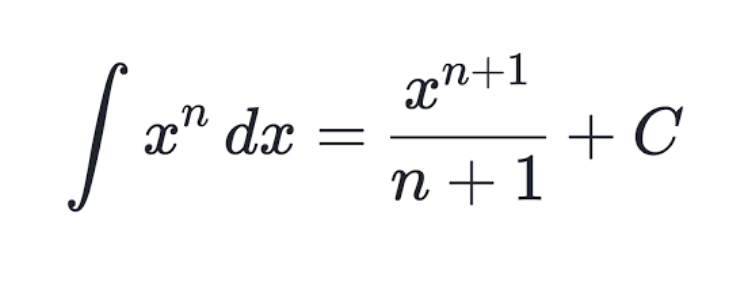top of page
Search

# Calculus at a Middle School Level(Cover Artist: Jacqueline Hill)

For most of my mathematics career, calculus seemed a distant field of math lacking the relevance and everydayness of classes like algebra or geometry – when’s the last time you’ve heard of a calculus word problem? After taking the course, it’s clear how much of math is influenced by calculus – from things like disease/population modeling to almost all of physics. With all this in mind, including the fact that this is going to skip over a lot of the more complicated algebra, I think it’s fair to say that calculus – the study of derivatives and integrals – can be broken down into a middle school level.

Firstly, what is a derivative? The most common definition is the rate of change, or slope. The slope of a line is equal to the m value in the form y = mx + b from the equation of a line – lines have a stable, unchanging slope, but a parabolic graph, for example, or most functions in general don’t have the same slope at all points. One way to find the slope at each of the points, the instantaneous slope (a more strict definition of the derivative, is to find the slope of the line that touches the graph at just that point, the tangent line.In the graph above, the slope at the point (x1, y1) of the parabola would be the positive value represented by the slope of the tangent line shown in blue. However, at the vertex of the parabola, the slope of the new tangent line would be a 0 because the line is flat, so obviously not the same value. Is there a more simple way to find the instantaneous slope of a function at any given point than long-winded algebra?

There is, by taking the derivative. The power rule of differentiation states that the instantaneous slope of any point on a function [xn] is equal to [n × x(n-1)].If you have a line with the equation y = 2x + 3, taking the derivative of the right side will yield [2 × 1× x(1-1)] or just 2, because the derivative of any constant value like 3 is 0. That should make sense, because, as stated earlier, the slope of any point on a line is just the slope of the line, which is the m value in y = mx + b.

For something like the parabola above, let’s say it has the equation y = x2 + 4. Then the derivative would be the exponent (2) multiplied by x(2-1), or 2x – the slope of any point on the function can be determined by simply multiplying the x-coordinate by 2.

The derivative links 3 of the most important functions in physics together – displacement/position, velocity, and acceleration. Displacement merely means distance from a point, like 2 meters. Velocity is the derivative of displacement, meaning it is the rate of change of the displacement – how fast does the displacement change every second (e.g., 2 meters/second)? Finally, acceleration is the derivative of velocity, meaning it is the rate of change of the velocity.How could you go from the acceleration back to velocity, and then back to displacement? The opposite of the derivative is integration, or the area under a curve. The symbol for it is “∫”, and the formula is essentially the opposite of the derivative formula – in the derivative, you multiplied the exponent with the coefficient of the x and decreased the original exponent by 1. In the integral, you add 1 to the exponent of the x and also divide the whole expression with the new exponent, and then add by some constant c.For example, the integral of 3x would be 1.5x2. If you want to take the integral or find the area under the graphs above, you can see that the position and acceleration graphs go all the way to infinity, so the area is not going to be a real/calculable number. That’s why to find the integrals, you have upper and lower bounds for the x value, to essentially restrict the domain so that the area under the curve is calculable.

Now that you know the basics, the applications of calculus are manifold. One of the most important real-world applications is in architecture and building. For example, if you want to determine how much cement is required to build a certain shape, like a bridge, you might need to use integration. Another famous example of calculus in architecture is with the Eiffel Tower – its creator, using calculus, had to take into account wind speeds at the top to minimize the effects on the tower, resulting in the equation for the shape of the tower.

Another real world application of calculus is in modeling – for example, the SIR model uses differential equations (a more complicated form of calculus) to help determine how dangerous diseases are, taking into account the rate of change of the Susceptible, Infected, and Recovered populations. A version of this model has been adapted for COVID-19 modeling.

So the next time you think about taking Calculus in the future, don’t be too anxious about it – it’s a powerful discipline of math that is well-rooted in algebra you already know, but linked to so many professions and disciplines of STEM that it’s exciting to see where it will show up again.

Bibliography

“Elegant Shape of Eiffel Tower solved Mathematically.” Phys.org. January 6, 2005

https://phys.org/news/2005-01-elegant-eiffel-tower-mathematically.html

“Power rule: How to w/ 9+ step by step examples!”. Calc Workshop. February 15, 2021.

https://calcworkshop.com/derivatives/power-rule/ (image source)

Beira, Maria Jardim and Sebastiao, Pedro Jose. “A differential equations model-fitting

analysis of COVID-19 epidemiological data to explain multi-wave dynamics.” Nature.com.

August 11, 2021.

https://www.nature.com/articles/s41598-021-95494-6

Dusto, Amy. “Motion Graphs: Position, Velocity, & Acceleration (w/ Diagram).”

Sciencing. December 28, 2020.

https://sciencing.com/motion-graphs-position-velocity-acceleration-w-diagram-13720230.ht

ml (image source)

“Find equation of Tangent to Parabola.” onlinemath4all.com.